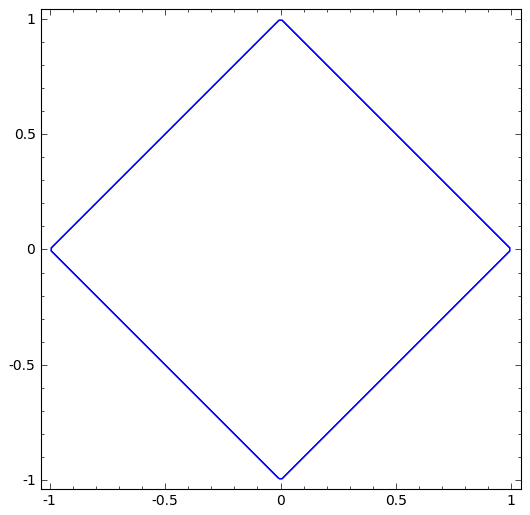# Simple volume calculation problem (double integrals)

[EDIT]: Found the mistake, see the next post.

## Homework Statement

Evaluate $$\iint_{S}{\rm e}^{x+y}dx\, dy,S=\{(x,y):\left|x\right|+\left|y\right|\leq1\}$$

2. The attempt at a solution
##\left|x\right|+\left|y\right|## is the rhombus with the center at the origin, symmetrical about both axes, so we find the volume at the first quadrant and then multiply by four.##\varphi(x)=1-x## limits the region of integration and so we have, first integrating over ##y## : $$\iint_{S}{\rm e}^{x+y}dx\, dy=4\int_{0}^{1}\left[\int_{0}^{1-x}{\rm e}^{x}{\rm e}^{y}dy\right]dx=1\neq {\rm e}-{\rm e}^{-1}$$ (I know the correct answer which is ##{\rm e}-{\rm e}^{-1}## )

Apparently so simple, but I just can't see the mistake.

Last edited:

Oh Lord, ##{\rm e}^{x+y}## obviously isn't symmetric.

LCKurtz
Homework Helper
Gold Member
Oh Lord, ##{\rm e}^{x+y}## obviously isn't symmetric.

True. Did you work the problem using the "obvious" change of variables?

True. Did you work the problem using the "obvious" change of variables?

Hrm... I guess I cheated: I took the total volume to be the sum of volumes over the triangles formed by the axes and four diagonal lines (##x+1##, ##1-x##, ##-x-1##, ##x-1##): $$\int_{-1}^{0}\left[\int_{0}^{x+1}{\rm e}^{x+y}dy\right]dx+\int_{-1}^{0}\left[\int_{-x-1}^{0}{\rm e}^{x+y}dy\right]dx+\ldots,$$ took the first one $$\int_{1}^{0}\left[\int_{0}^{x+1}{\rm e}^{x+y}dy\right]dx=\int_{-1}^{0}{\rm e}^{2x+1}-{\rm e}^{x}dx=\frac{1}{2}({\rm e}+{\rm e}^{-1})-1$$ and then asked sage to do the rest because they are essentially the same and evaluating them all has no didactic value.

By the way, I'm learning this stuff on my own, so I might not know the standard way of approaching problems like this. My book (Apostol) says nothing about the change of variables if I understand correctly.

What I dislike about this sort of examples is that I have to plot some of the functions first to actually understand what's going; TBH I'd enjoyed more analytic (or algorithmic) approach, where the peculiarities of a particular example wouldn't matter.

(too many "I"'s in this post)

HallsofIvy
Homework Helper
No, that's not what LCKurtz meant by "the obvious change of variables".

If you let u= x+ y and v= x- y, then x= (u+ v)/2 and y= (u- v)/2. The figure is then $-1\le u\le 1$, $-1\le v\le 1$.The Jacobean is 1/2 so the integral becomes
$$\frac{1}{2}\int_{u=-1}^1\int_{v=-1}^1 e^u dvdu$$

No, that's not what LCKurtz meant by "the obvious change of variables".

I never suggested that.

Change of variables is some ten subsections below my current position in Apostol, but I liked the technique, thank you.